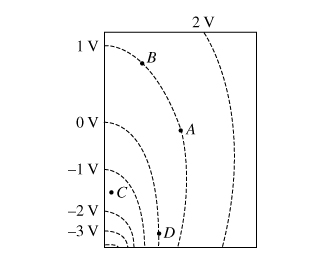# Problem: The dashed lines in the diagram represent cross sections of equipotential surfaces drawn in 1-V increments.What is the work WAD done by the electric force to move a 1-C charge from A to D?

###### FREE Expert Solution

Work:

$\overline{){\mathbf{W}}{\mathbf{=}}{\mathbf{-}}{\mathbf{∆}}{\mathbf{U}}}$

Change in potential difference:

87% (300 ratings)###### Problem Details

The dashed lines in the diagram represent cross sections of equipotential surfaces drawn in 1-V increments.What is the work WAD done by the electric force to move a 1-C charge from A to D?